# e. public class Queue { // Uses the correct Stack class from ME2 Ex 19 private Stack mStack; public Queue() { setStack(new Stack()); } public Queue enqueue(E pData) { getStack().push(pData); return t...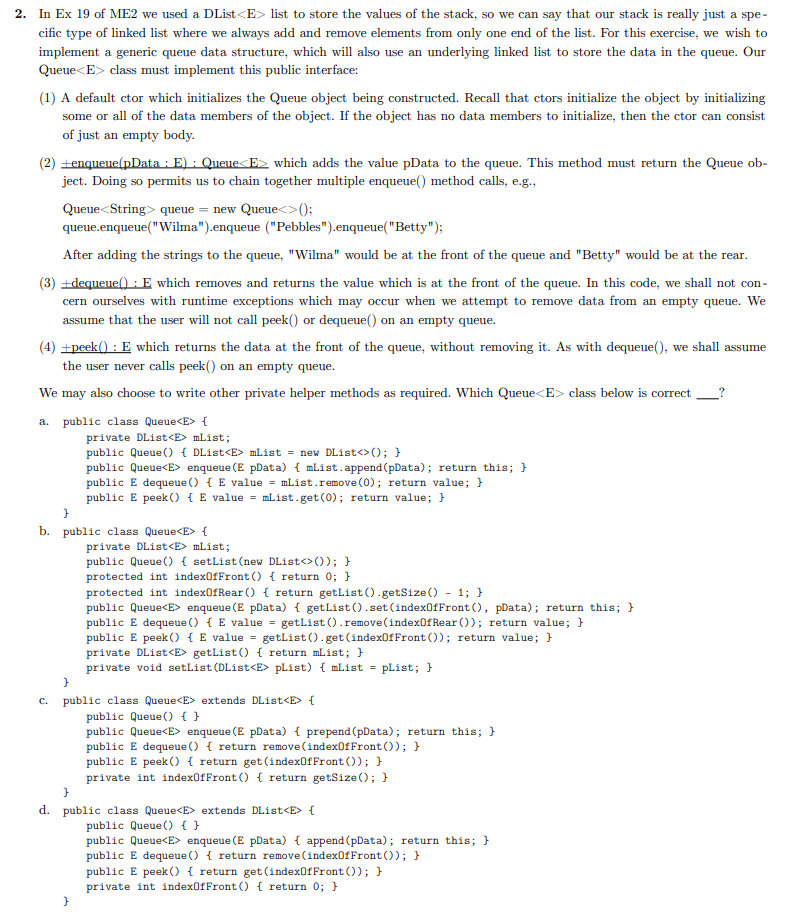e. public class Queue { // Uses the correct Stack class from ME2 Ex 19 private Stack mStack; public Queue() { setStack(new Stack()); } public Queue enqueue(E pData) { getStack().push(pData); return this; } public E dequeue() { return getStack().pop(); } public E peek() { return getStack.peek(); } private Stack getStack() { return mStack; } private void setStack(Stack pStack) { mStack = pStack; } }

f. public class Queue extends Stack { // Uses the correct Stack class from ME2 Ex 19 public Queue() { super(); } public Queue enqueue(E pData) { push(pData); return this; } public E dequeue() { return pop(); } public E peek() { return peek(); } }

2. In Ex 19 of ME2 we used a DList

Implementation of Option B is the correct one.

```DList.java

public class DList<E> {
//intialize the list

//get the size of the list
public int getSize(){
return list.size();
}

//remove the elment from the list
public E remove(int index){
return list.remove(index);
}

//set the element at index
public void set(int index, E value){
list.set(index,value);
}

//get the elemnt from the index
public E get(int index){
return list.get(index);
}
}
```
```Queue.java

public class Queue<E> {
private DList<E> mList;

public Queue(){
setList(new DList<>());
}

protected int indexOfFromt(){
return 0;
}

protected int indexOfRear(){
return getList().getSize() - 1;
}

public Queue<E> enqueue(E pData){
getList().set(indexOfFromt(),pData);
return this;
}

public E dequeue(){
E value = getList().remove(indexOfRear());
return value;
}

public E peek(){
E value = getList().get(indexOfFromt());
return value;
}

private DList<E> getList(){
return mList;
}

private void setList(DList<E> pList){
mList = pList;
}
}
```
##### Add Answer of: e. public class Queue { // Uses the correct Stack class from ME2 Ex 19 private Stack mStack; public Queue() { setStack(new Stack()); } public Queue enqueue(E pData) { getStack().push(pData); return t...
Similar Homework Help Questions
• ### JAVA PROGRAM: public class Stack { private DList mList; public Stack() { setList(new DList<>()); } private int indexOfTop() { return getList().isEmpty() ? -1 : getList().getSize() - 1; } public...

JAVA PROGRAM: public class Stack { private DList mList; public Stack() { setList(new DList<>()); } private int indexOfTop() { return getList().isEmpty() ? -1 : getList().getSize() - 1; } public E peek() { return getList().get(indexOfTop()); } public E pop() { return getList().remove(indexOfTop()); } public Stack push(E pData) { getList().append(pData); return this; } private DList getList() { return mList; } private void setList(DList pList) { mList = pList; } Q1 Because Stack encapsulates an instance variable which is of the class DList,...

• ### Java Code Class Queue

Modify the class Queue to include appropriate error messages if invalid conditions occur. For example, trying to dequeue an item when the queue is empty.Here is the Main://Week 6 - lecture: driver program (queue)public class Main{public static void main(String[] args){Queue q = new Queue();System.out.println("Insertion of 10 characters in q");for (int i = 0; i < 10; i++){int x = 32 + (int)(Math.random()*95);System.out.println(x + " --> " + (char)x);q.enqueue((char)x);}System.out.println("nDisplaying and deleting elements");for (int i = 0; i < 10; i++){System.out.println("Item at...

• ### AQueue.java class AQueue implements Queue { private E queueArray[]; // Array holding queue elements private static...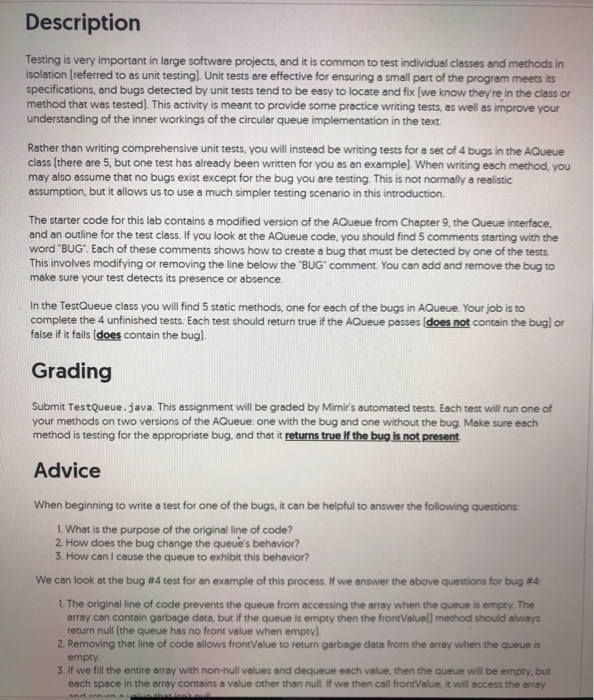AQueue.java class AQueue implements Queue { private E queueArray[]; // Array holding queue elements private static final int DEFAULT_SIZE = 10; private int maxSize; // Maximum size of queue private int front; // Index of front element private int rear; // Index of rear element // Constructors @SuppressWarnings("unchecked") // Generic array allocation AQueue(int size) { //BUG #1: maxSize = size maxSize = size+1; // One extra space is allocated rear = 0; front = 1; queueArray = (E[])new Object[maxSize]; //...

• ### Write Queue Class

1. Use a chain of Nodes to design and create a class Queue that implements the interface QueueInterface<String> having the following methods:• public void insert(E x); // E is interpreted as String in this and the following methods• public E remove(); // if the Queue is empty, this returns null and doesn’t change anything• public boolean empty();• public E peek();// if the Queue is empty, this returns null• public int size(); // you can just copy these method signatures to...

• ### 2. Consider a circular array based Queue as we have discussed in the lectures (class definition...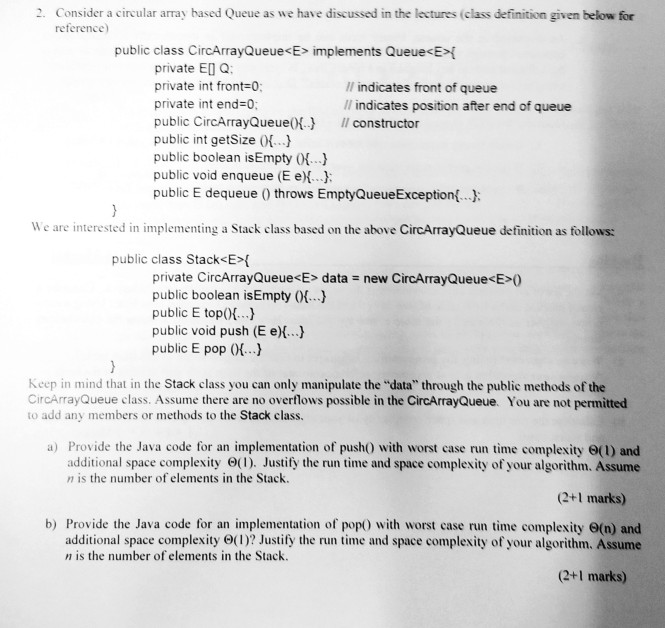2. Consider a circular array based Queue as we have discussed in the lectures (class definition given below for reference) public class CircArrayQueue<E> implements Queue<E private EI Q private int front-0 indicates front of queue l indicates position after end of queue private int end-0: public CircArrayQueue( public int getSize (.. public boolean isEmpty ( public void enqueue (E e)... public E dequeue ) throws EmptyQueueException... Il constructor We are interested in implementing a Stack class based on the above...

• ### JAVA Implement a MyQueue class which implements a queue using two stacks. private int maxCapacity...

JAVA Implement a MyQueue class which implements a queue using two stacks. private int maxCapacity = 4; private Stack stack1; private Stack stack2; Note: You can use library Stack but you are not allowed to use library Queue and any of its methods Your Queue should not accept null or empty String or space as an input You need to implement the following methods using two stacks (stack1 & stack2) and also you can add more methods as well: public...

• ### Array-based Queue Lecture 6 Two Class Exercises | Class Exercise #1 - Create an array-based queue that holds value...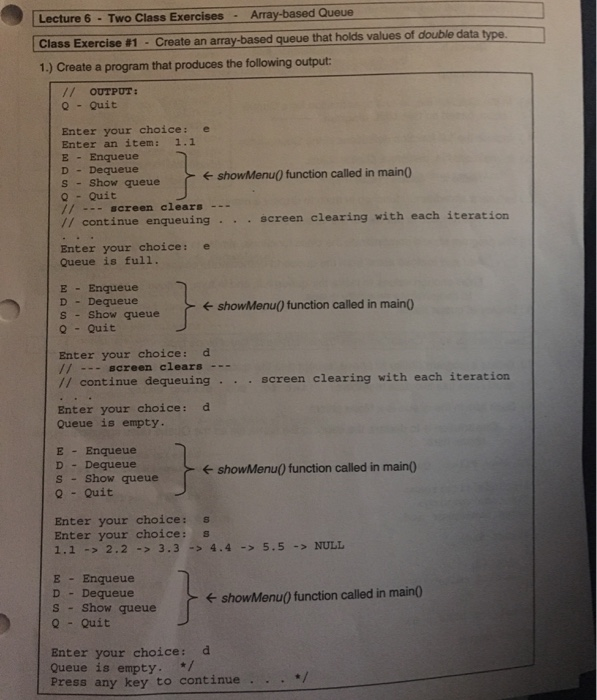Array-based Queue Lecture 6 Two Class Exercises | Class Exercise #1 - Create an array-based queue that holds values of double data type. 1.) Create a program that produces the following output OUTPUT: Q Quit Enter your choice: e Enter an item: 1.1 E Enqueue D Dequeue s-show queue ← showMenuO function called in main) OQuit // screen clears-.. continue enqueuing.screen clearing with each iteration Enter your choice: e Queue is full. E Enqueue D Dequeue s Show queue 0...

• ### In java Build a QueueInt class for integers that is compatible with the driver code below. The QueueInt should operate in a FIFO (first in, first out) fashion and implement the variables and methods a...

In java Build a QueueInt class for integers that is compatible with the driver code below. The QueueInt should operate in a FIFO (first in, first out) fashion and implement the variables and methods also listed below: Data Members: Declare and initialize, as needed, the data item(s) you will need to manage a queue of integers. You may only use arrays and primitives for your instance and/or static variables (I,e You can’t use Java defined Queue / Stack / List...

• ### To solve real world problem, the programer uses ADT like list, stack, queue, set, map, ... etc. t...

To solve real world problem, the programer uses ADT like list, stack, queue, set, map, ... etc. to build our data model. In AS8, we pick and choose STL queue and stack to build a postfix calculator. In this assignment you are to Design your own linked-list, a series of integer nodes. The private attributes of this IntLinked Queue including a integer node struct and private control variables and methods: private: struct Node { int data; Node *next; }; Node...

• ### Answer this plz with java lang. Question is : Here is the class you will use public interface Stack<E> {    /**    * Returns the number of elements in the stack.    * @return number of elements...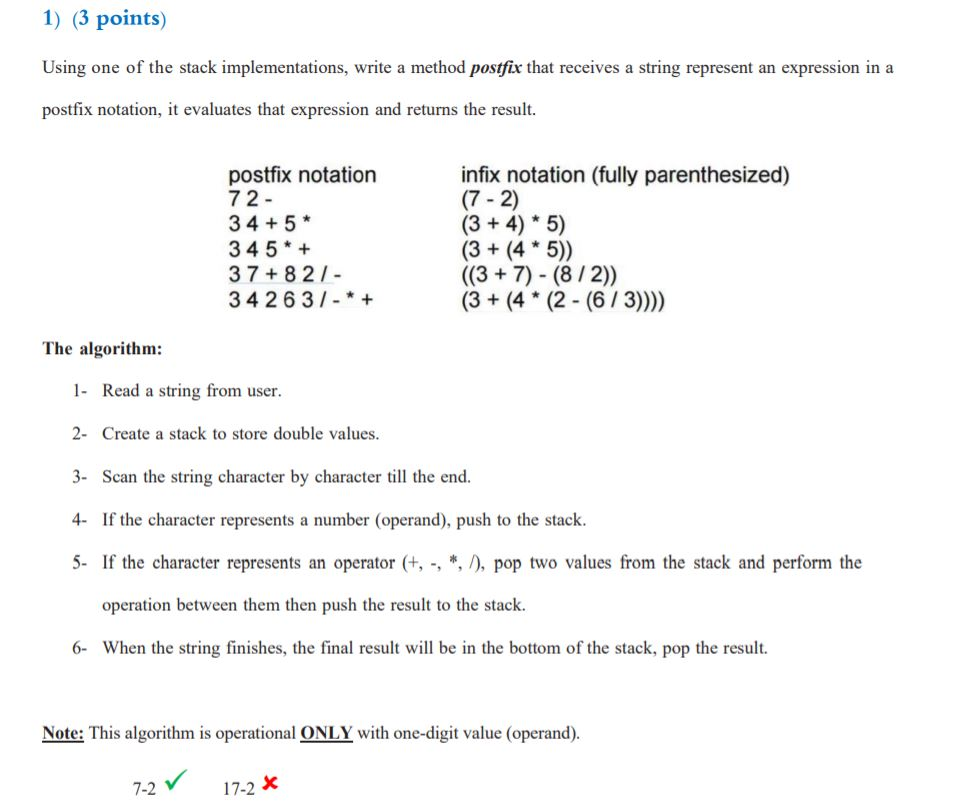Answer this plz with java lang. Question is : Here is the class you will use public interface Stack<E> {    /**    * Returns the number of elements in the stack.    * @return number of elements in the stack    */    int size( );    /**    * Tests whether the stack is empty.    * @return true if the stack is empty, false otherwise    */    boolean isEmpty( );    /**    * Inserts an element at the top of the stack.    * @param e the element...

Need Online Homework Help?